# Find 3d distance between 2 sets of points

2058
21
12-05-2016 02:21 AMby
New Contributor

Hi,

I'd like to find the 3d distance between all points in two point feature classes. One is 12 000 building points, the other 22 000 point locations of a construction machine (along a line), constructing many km of tunnel. My aim is to be able to select the building points within x meters when the construction machine is at a specific location. How can I do this without running Near 3d several thousand times?

I am using arcgis 10.3 with basic license. I am not very familiar with scripting.

Tags (3)
1 Solution

Accepted SolutionsbyEsri Esteemed Contributor

As an alternative I generated a script that will calculate the 3D distance between two sets of 3D point featureclasses.

The result is a table that contains the OID of the buildings, the OID of the tunnel points and the 3D distance:By defining two relates in ArcMap you will be able to do what you are looking for.

Relate 1: based on the tunnel points, relating to the new table.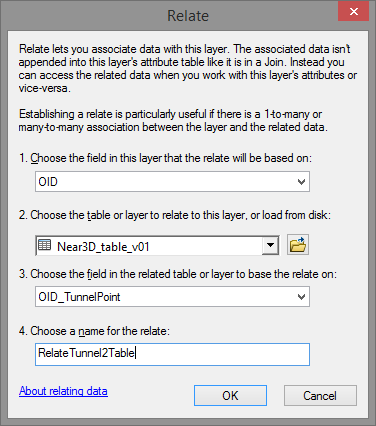Relate 2: based on the table relating to the building points:Make sure to define some attribute indexes in the table to increase the performance: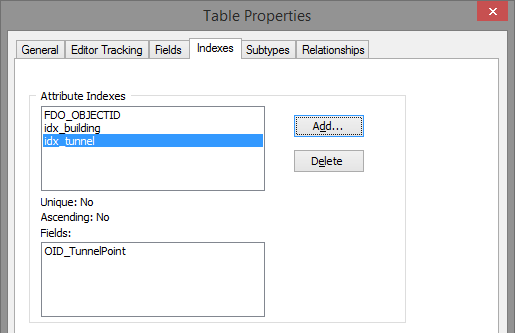How does it work. In ArcMap you select a tunnel point and open the attribute table of the tunnel point featureclass: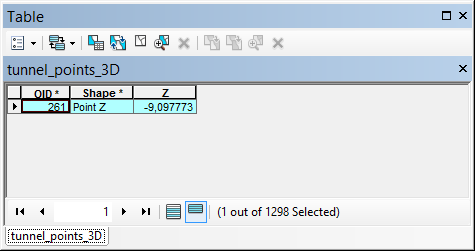Click on the relate button and select the relate: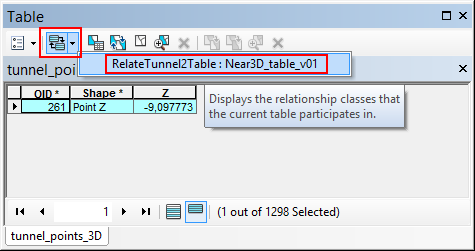This will open the table with the related combinations selected: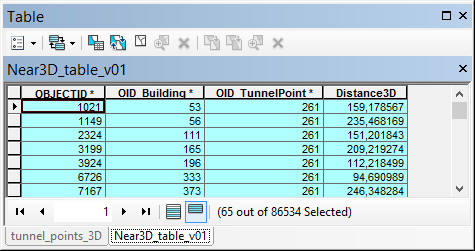Then select the relate to propagate the selection to the building points: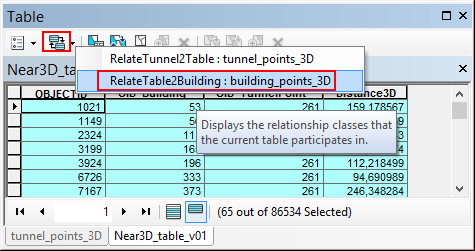... which will open the selected building points:The map will show the selection: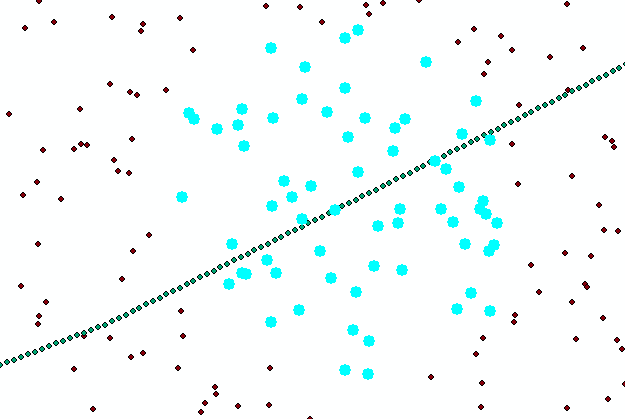The script I used for this is listed below. In my case I only had 6.5M combinations and it took 2 minutes to process the combinations on an average computer. This will probably take all night processing the 264M combinations.

Script:

``import mathimport arcpyfrom time import localtime, strftimedef main():    import os    ShowProgress("Start process...")    fc_buildings = r'D:\Xander\GeoNet\Generate3DNearTable\gdb\data.gdb\building_points_3D'    fc_tunnel_points = r'D:\Xander\GeoNet\Generate3DNearTable\gdb\data.gdb\tunnel_points_3D'    tbl_out = r'D:\Xander\GeoNet\Generate3DNearTable\gdb\data.gdb\Near3D_table_v01'    fld_building_id = 'OID_Building'    fld_tunnel_point_id = 'OID_TunnelPoint'    fld_dist3D = 'Distance3D'    # max distance    max_dist = 250    # sr = arcpy.Describe(fc_buildings).spatialReference    # create lists of buildings and tunnel points    ShowProgress("Create lists of buildings and tunnel points...")    flds = ('OID@', 'SHAPE@')    lst_building = [[r, r] for r in arcpy.da.SearchCursor(fc_buildings, flds)]    lst_tunnelpoints = [[r, r] for r in arcpy.da.SearchCursor(fc_tunnel_points, flds)]    # create output table for relate    ShowProgress("Create output table...")    tbl_ws, tbl_name = os.path.split(tbl_out)    arcpy.CreateTable_management(tbl_ws, tbl_name)    ShowProgress("Add fields to output table...")    AddField(tbl_out, fld_building_id, "LONG", 8)    AddField(tbl_out, fld_tunnel_point_id, "LONG", 8)    AddField(tbl_out, fld_dist3D, "DOUBLE", 8)    cnt_total = len(lst_building) * len(lst_tunnelpoints)    flds = (fld_building_id, fld_tunnel_point_id, fld_dist3D)    ShowProgress("Start creating combinations...")    with arcpy.da.InsertCursor(tbl_out, flds) as curs:        cnt = 0        cnt_found = 0        for building_data in lst_building:            oid_building = building_data            pntg_building = building_data            for tunnel_data in lst_tunnelpoints:                oid_tunnel_point = tunnel_data                pntg_tunnel_point = tunnel_data                cnt += 1                if cnt % 10000 == 0:                    ShowProgressPercentage("Processing combination: {0},  found: {1}".format(cnt, cnt_found), cnt, cnt_total)                dist3D = get3Ddistance(pntg_building, pntg_tunnel_point)                if dist3D <= max_dist:                    cnt_found += 1                    row = (oid_building, oid_tunnel_point, dist3D, )                    curs.insertRow(row)    ShowProgressPercentage("Ready processing {0} combinations,  found: {1}".format(cnt, cnt_found), cnt, cnt_total)def AddField(fc, fld_name, fld_type, fld_length):    if len(arcpy.ListFields(fc, fld_name)) == 0:        arcpy.AddField_management(fc, fld_name, fld_type, None, None, fld_length)def get3Ddistance(pntg1, pntg2):    pnt1 = pntg1.firstPoint    pnt2 = pntg2.firstPoint    distance = math.sqrt((pnt1.X-pnt2.X)**2 + (pnt1.Y-pnt2.Y)**2 + (pnt1.Z-pnt2.Z)**2)    return distancedef ShowProgressPercentage(txt, cnt, cnt_tot):    current_time = strftime("%H:%M:%S", time.localtime())    if cnt_tot is None:        print "{0}   - {1}".format(current_time, txt)    else:        percentage = float(cnt) * 100.0 / float(cnt_tot)        print "{0}   - {1} ({2}%)".format(current_time, txt, round(percentage, 2))def ShowProgress(txt):    # current_time = strftime("%Y-%m-%d %H:%M:%S", gmtime())    current_time = strftime("%H:%M:%S", time.localtime())    print "{0} - {1}".format(current_time, txt)if __name__ == '__main__':    main()‍‍‍‍‍‍‍‍‍‍‍‍‍‍‍‍‍‍‍‍‍‍‍‍‍‍‍‍‍‍‍‍‍‍‍‍‍‍‍‍‍‍‍‍‍‍‍‍‍‍‍‍‍‍‍‍‍‍‍‍‍‍‍‍‍‍‍‍‍‍‍‍‍‍‍‍‍‍‍‍‍‍‍‍‍‍‍‍‍‍‍``
21 RepliesbyEsri Esteemed Contributor

The Near 3D—Help | ArcGIS for Desktop tool will get you the distance to the nearest object in the other layer, not all objects. The Generate Near Table—Help | ArcGIS for Desktop will calculate the distance between all objects in two featureclasses, but not in 3D.

I suppose you would have to script this, but this means evaluating 12000 x 22000 = 264M combinations. That will be a pretty long process. Also to work with the results will be slow, since your table will have 264M records.

To calculate the 3D distance between is not very complex. Just take the square root from (dx² + dy² + dz²).by
New Contributor

Thanks Xander. I guess the easiest solution is to run Near 3D after specifying one location of the construction machine in the first feature class. My aim was to have this available for my colleagues to query from a table, but there would be too much data.byEsri Esteemed Contributor

Just wondering... is there a lot of difference in Z between the building points and the construction machine points?

The fact that you will limit the search distance can limit a lot the number of results. Also if you apply a "Relate" and define your attribute indexes correctly, this might yield be a workable solution.

Is it possible to share your data, to see what's can be achieved?

Kind regards, Xanderby
New Contributor

The Z difference is between 5 and 100 meters.

Limiting the search radius would at the most cut the number of building points in two, leaving me with 6000 points.The building points are all within 400 meters of the line that the construction machine points run along, and I would need to select building points within 150-250 meters from each construction machine point.

I unfortunately cannot share the data.

Thanks again Xander.byEsri Esteemed Contributor

The search radius that I was referring to is for searching the buildings for each point on the tunnel line. As you mention that you only want to consider buildings up to 250 m from a tunnel point, this will mean that you will not have to generate the 264M combinations.

I will come back to this and see if I can think of an easy way to generate a result using a Basic license.by
New Contributor

Very much appreciated.

I am in the process of trying out the following solution, but not quite sure about it:

I ran 3D buffer tool for each construction machine point and got 22 000 multipatch 200 meter buffer spheres. I then ran Inside 3D to get a table with all the building points within each buffer sphere/multipatch (which had to be joined to the two point tables to preserve all my information in one table). In the resulting table (only 1 million rows) I have xyz for each construction machine point (=the center of the 3D buffer multipatch) and xyz for each building point, can I calculate 3D distance using the coordinates?byMVP Honored Contributor

You could use Generate Near Table to calculate the 2d distances ('c' below), then Spatial Join to transfer start/end height attribute (difference is 'd' below), then calculate the 3d distance to a new field ('e' below).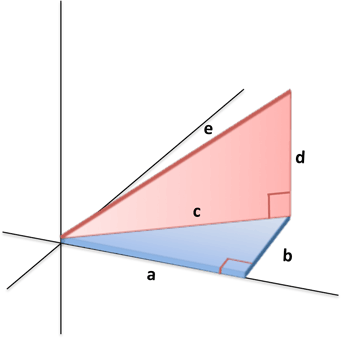by
New Contributor

Thanks, this seems interesting. I unfortunately only have the basic license and need advanced to use Generate Near Table.by
New Contributor

Hi again,

As mentioned in a comment to Xander above, I used 3D Buffer and Inside 3D plus some table joining to end up with a table with xyz coordinates for both building points and construction machine points. Can you tell me how to calculate 3D distance in a new field, either using excel or in the attribute table?

This is an example (with very many zeros for some reason)

 POINT_X_building POINT_Y_building POINT_Z_building POINT_X_constructionmachineno2850 POINT_Y_constructionmachineno2850 POINT_Z_constructionmachineno2850 599017.980859000000000 6640584.964650000400000 123.000000000000000 599075.213999999690000 6640639.921700000800000 -6.040000000000000 598973.976436999980000 6640633.025369999900000 121.950400000000000 599075.213999999690000 6640639.921700000800000 -6.040000000000000 598973.976436999980000 6640633.025369999900000 121.950400000000000 599075.213999999690000 6640639.921700000800000 -6.040000000000000 599111.963903000000000 6640589.985279999700000 126.000000000000000 599075.213999999690000 6640639.921700000800000 -6.040000000000000 599090.217955000000000 6640602.671959999900000 126.000000000000000 599075.213999999690000 6640639.921700000800000 -6.040000000000000